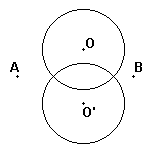# Cross a Circle by a Line Segment

### Geometric Construction with the Compass Alone

A segment AB is given by its end points A and B. A circle is given by its radius R and the center O. Assume O does not lie on AB. Find the points of intersection of the circle with the segment AB.## Solution

You should tackle the second problem before trying this one.

First of all, using the second problem construct the point O' symmetric to O with respect to AB. Then draw a circle centered at O' with the radius R. If the two circles intersect, the points of intersection lie on the line AB and, therefore, serve as points of intersection of the original circle with AB. In this case we have two solutions. If the circles just touch each other, the solution is unique. In the last case, where the circles have no points in common, the problem has no solution.### Problems (Use a compass only)